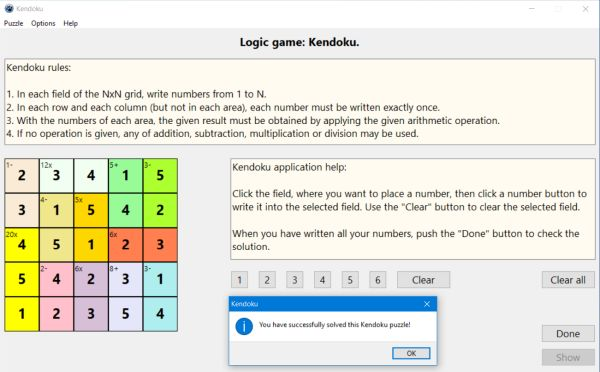# Computing: Free Pascal Programming

## Logic number puzzle: Kendoku (KenKen, CalcuDoku).

Description: Puzzle game with numbers to be placed onto a grid. The rules of the game are as follows:
1. In each field of the NxN grid, write numbers from 1 to N.
2. In each row and each column (but not in each area), each number must be written exactly once.
3. With the numbers of each area, the given result must be obtained by applying the given arithmetic operation.
4. If no operation is given, any of addition, subtraction, multiplication or division may be used.
The game is a typical logic game: In order to find the correct solution of the puzzle, you have to explore the arithmetic operations and area results given and the numbers already written, and by logical thinking (concluding from what you know, that a given field must be a given number, or on the contrary, can't be a given number), determine, which fields have to contain which numbers.
The game is played exclusively with the mouse: Click the field, where you want to write a number, then click one of the number buttons to write the corresponding number or Clear to remove the number from the actually selected field.
Note: Kendoku may be considered as an extended combination of Sumdoku (area sums) and Factors (area products).

To do: The fourth rule isn't implemented in the actual version of the puzzle (there is an arithmetic operation given for each area).

Free Pascal features: Changing the color of shapes and the caption of static-texts during runtime. Using buttons to insert characters into a given control's caption. Two-dimensional arrays, arrays of records (classic Pascal).

Screenshot: